JEE  >  Test: Comprehension Based Questions: Applications of Derivatives

# Test: Comprehension Based Questions: Applications of Derivatives

Test Description

## 7 Questions MCQ Test Maths 35 Years JEE Main & Advanced Past year Papers | Test: Comprehension Based Questions: Applications of Derivatives

Test: Comprehension Based Questions: Applications of Derivatives for JEE 2023 is part of Maths 35 Years JEE Main & Advanced Past year Papers preparation. The Test: Comprehension Based Questions: Applications of Derivatives questions and answers have been prepared according to the JEE exam syllabus.The Test: Comprehension Based Questions: Applications of Derivatives MCQs are made for JEE 2023 Exam. Find important definitions, questions, notes, meanings, examples, exercises, MCQs and online tests for Test: Comprehension Based Questions: Applications of Derivatives below.
Solutions of Test: Comprehension Based Questions: Applications of Derivatives questions in English are available as part of our Maths 35 Years JEE Main & Advanced Past year Papers for JEE & Test: Comprehension Based Questions: Applications of Derivatives solutions in Hindi for Maths 35 Years JEE Main & Advanced Past year Papers course. Download more important topics, notes, lectures and mock test series for JEE Exam by signing up for free. Attempt Test: Comprehension Based Questions: Applications of Derivatives | 7 questions in 10 minutes | Mock test for JEE preparation | Free important questions MCQ to study Maths 35 Years JEE Main & Advanced Past year Papers for JEE Exam | Download free PDF with solutions
 1 Crore+ students have signed up on EduRev. Have you?
Test: Comprehension Based Questions: Applications of Derivatives - Question 1

### PASSAGE - 1 If a continuous function f defined on the real line R, assumes positive and negative values in R then the equation f(x) = 0 has a root in R. For example, if it is known that a continuous function f on R is positive at some point and its minimum value is negative then the equation f(x) = 0 has a root in R. Consider f(x) = kex – x for all real x where k is a real constant. Q. The line y = x meets y = kex for k < 0 at

Detailed Solution for Test: Comprehension Based Questions: Applications of Derivatives - Question 1

For k = 0, line y = x meets y = 0, i.e., x-axis only at one point.
For k < 0, y = kex meets y = x only once as shown in the graph.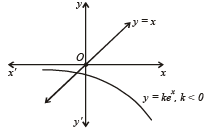Test: Comprehension Based Questions: Applications of Derivatives - Question 2

### PASSAGE - 1 If a continuous function f defined on the real line R, assumes positive and negative values in R then the equation f(x) = 0 has a root in R. For example, if it is known that a continuous function f on R is positive at some point and its minimum value is negative then the equation f(x) = 0 has a root in R. Consider f(x) = kex – x for all real x where k is a real constant. Q. The positive value of k for which kex – x = 0 has only one root is

Detailed Solution for Test: Comprehension Based Questions: Applications of Derivatives - Question 2

Let f (x) = kex – x

Now for f (x) = 0 to have only one root means the line y = x must be tangential to the curve y = kex.
Let it be so at (x1, y1) then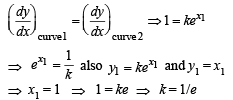Test: Comprehension Based Questions: Applications of Derivatives - Question 3

### PASSAGE - 1 If a continuous function f defined on the real line R, assumes positive and negative values in R then the equation f(x) = 0 has a root in R. For example, if it is known that a continuous function f on R is positive at some point and its minimum value is negative then the equation f(x) = 0 has a root in R. Consider f(x) = kex – x for all real x where k is a real constant. Q. For k > 0, the set of all values of k for which kex – x = 0 has two distinct roots is

Detailed Solution for Test: Comprehension Based Questions: Applications of Derivatives - Question 3

∵ For y = x to be tangent to the curve y = kex, k = 1/e
∴ For y = kex to meet y = x at two points we should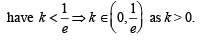Test: Comprehension Based Questions: Applications of Derivatives - Question 4

PASSAGE - 2

Let f (x) = (1 – x)2 sin2x + x2 for all x ∈ I R and let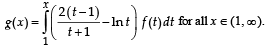Q.

Consider the statements:

P : Th er e exists some x ∈ R such th at f (x) + 2 x =  2(1 + x2)

Q : There exists some x ∈ R such that 2 f (x) + 1 = 2x (1 + x) Then

Detailed Solution for Test: Comprehension Based Questions: Applications of Derivatives - Question 4

For the statement P
f(x) + 2x = 2 (1 + x2)
⇒ (1 – x)2 sin2x + x2 + 2x = 2(1+ x2)
⇒ (1 – x)2 sin2x = x2 – 2x + 1 + 1
⇒ (1 – x)2 sin2x = (1 – x)2 + 1
⇒ (1 – x)2 cos2x = –1

Which is not possible for any real value of x.
∴ P is not true.
Also let H(x) = 2f(x) + 1 – 2x (1 + x)
H(0) = 2f(0) + 1 – 0 = 1
and H(1) = 2f(1) + 1 – 4 = – 3
⇒ H(x) has a solution in (0, 1)
∴ Q is true.

Test: Comprehension Based Questions: Applications of Derivatives - Question 5

PASSAGE - 2

Let f (x) = (1 – x)2 sin2x + x2 for all x ∈ I R and letQ. Which of the following is true?

Detailed Solution for Test: Comprehension Based Questions: Applications of Derivatives - Question 5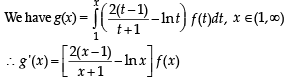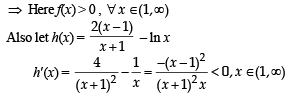∴ h(x) is decreasing function.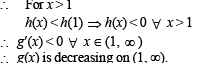Test: Comprehension Based Questions: Applications of Derivatives - Question 6

PASSAGE - 3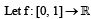(the set of all real numbers) be a function. Suppose the function f is twice differentiable, f(0) = f(1) = 0 and satisfies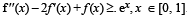Q. Which of the following is true for 0 < x < 1?

Detailed Solution for Test: Comprehension Based Questions: Applications of Derivatives - Question 6

We have f ''(x) – 2f '(x) +f(x) > ex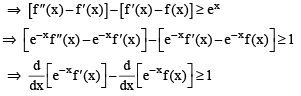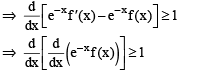Let  g(x) = e–xf(x)
Then we have g ''(x) > 1> 0
So g is concave upward.
Also g(0) = g(1) = 0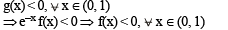Test: Comprehension Based Questions: Applications of Derivatives - Question 7

PASSAGE - 3(the set of all real numbers) be a function. Suppose the function f is twice differentiable, f(0) = f(1) = 0 and satisfiesQ. If the function e–x f(x) assumes its minimum in the interval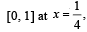which of the following is true?

Detailed Solution for Test: Comprehension Based Questions: Applications of Derivatives - Question 7

g(x) = e–xf(x)
⇒ g'(x) = e–xf '(x) – e–xf(x) = e–x (f '(x) – f(x))
As = 1/4 is point of local minima in [0, 1]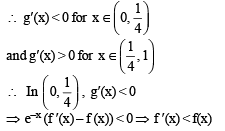## Maths 35 Years JEE Main & Advanced Past year Papers

132 docs|70 tests
 Use Code STAYHOME200 and get INR 200 additional OFF Use Coupon Code
Information about Test: Comprehension Based Questions: Applications of Derivatives Page
In this test you can find the Exam questions for Test: Comprehension Based Questions: Applications of Derivatives solved & explained in the simplest way possible. Besides giving Questions and answers for Test: Comprehension Based Questions: Applications of Derivatives, EduRev gives you an ample number of Online tests for practice

## Maths 35 Years JEE Main & Advanced Past year Papers

132 docs|70 tests